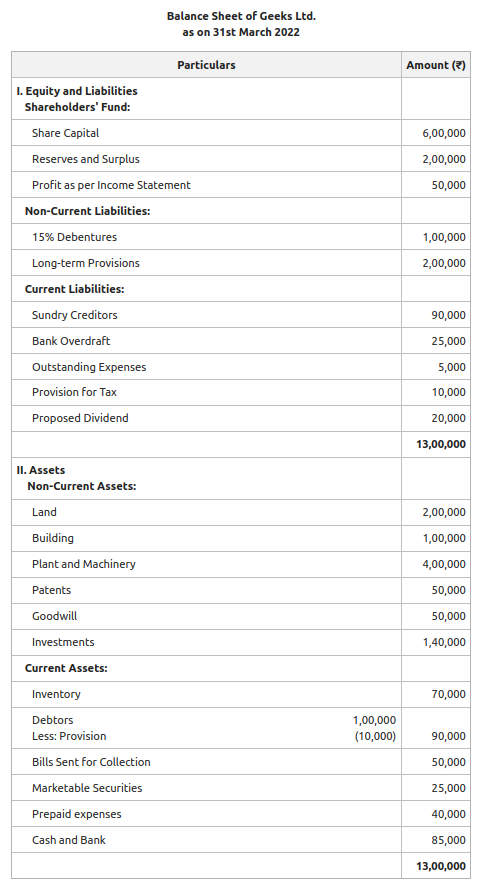Open in App
Not now

# Liquid/Quick Ratio: Meaning, Formula, Significance and Examples

• Last Updated : 07 Sep, 2022

Financial Statements are prepared to know the profitability and financial position of the business in the market. These financial statements are then analysed with the help of different tools and methods. Ratio Analysis is one of the methods to analyse financial statements. The relationship between various financial factors of a business is defined through ratio analysis.

Liquid ratio is the one that is used to derive a relation between the liquid assets and current liabilities of a firm. It is used to determine whether the liquid assets lying with a firm would be sufficient to pay off its current obligations or not. In other words, it is used to depict the magnitude of liquid assets against the current liabilities of a concern. It is also called the quick ratio or acid-test ratio.

Liquid ratio is one of the two main liquidity ratios. Liquidity ratios are the ones that determine the organisation’s ability to meet its short-term obligations by defining a systematic relationship between the amount of current/liquid assets and that of its current/short-term obligations.

Generally, a liquid ratio of 1:1 is considered ideal, which means that the liquid assets should just be enough to cover the amount of current liabilities. An organization may or may not be able to maintain this ideal ratio at all times in the accounting period. However, necessary steps should be taken, so as to not deflect too much from the ideal value.

• Liquid Assets: Such assets include cash and equivalents and other such assets, which can be converted by the organization into cash with minimal or no discounting. In other words, quick assets are not held by the firm with the purpose of capitalisation at all.  Examples: Cash, Cash Equivalents, Accounts Receivable, Marketable Securities, etc.
• Current Liabilities: This refers to the amounts payable to the firm’s creditors and lenders within a period of one year or within the standard operating cycle, whichever is shorter. They are typically settled using the firm’s current assets. Examples: Accounts payable, short-term debts like commercial paper, current maturity of long-term loans, income tax due for the year, etc.

### Formula:where,

Liquid Assets = Cash  + Accounts Receivable + Short-term Investments (Marketable Securities)

or

Liquid Assets = Current Assets – Inventory – Prepaid Expenses

Current Liabilities = Bank Overdraft + Trade Payables + Provision of Taxation + Proposed Dividends + Unclaimed Dividends + Outstanding Expenses + Loans Payable within a Year

#### There can be three situations arising from the calculation:

If Liquid Assets > Current Liabilities, then Quick Ratio > 1: This implies that the organisation would still have some assets left even after paying all the short-term debts, and is a desirable situation to be in.

If Liquid Assets = Current Liabilities, then Quick Ratio = 1:  This means that the quick assets are just enough to cover the short-term obligations of the firm, and none would be left after all settlements.

If Liquid Assets < Current Liabilities, then Quick Ratio < 1: This is not an ideal situation to be in since it implies that the company does not have enough resources to pay off short-term debts.

### Significance:

Liquid Ratio is computed to know the ability of a firm to pay off the short-term liabilities of a firm with the help of liquid assets. In other words, this ratio is calculated to determine the short-term solvency of a firm. 1:1 is considered an ideal liquid ratio. That means the liquid assets should be equal to the current liabilities of the firm. But if the liquid ratio is very high, it is believed that the funds are lying idle. Creditors use the quick ratio of a firm to evaluate the creditworthiness of the business and to determine whether to advance loans to such a firm or not. It also acts as a complementary measure to the current ratio of the firm.

Illustration 1:

Calculate the quick ratio from the following balance sheet of Geeks Ltd. for the year ending March 2022:Solution:

Liquid Assets = Debtors + Bills sent for collection + Marketable Securities + Cash and Bank

= 90,000 + 50,000 + 25,000  + 85,000

= ₹2,50,000

Current Liabilities = Bank Overdraft + Sundry Creditors + Outstanding Expenses + Provision for Tax + Proposed Dividend

= 90,000 + 25,000 + 5,000 + 10,000 + 20,000

= ₹1,50,000

Now,Liquid Ratio= 1.67:1

Illustration 2:

Calculate the quick ratio from the following information:

Current Assets ₹90,000; Inventory ₹12,000; Working Capital ₹60,000.

Solution:

Quick Assets = Current Assets – Inventory

= 90,000 – 12,000

= ₹78,000

Working Capital = Current Assets – Current Liabilities

60,000 = 90,000 – Current Liabilities

Thus, Current Liabilities = ₹30,000

Now,Quick Ratio = 2.6:1

My Personal Notes arrow_drop_up
Related Articles